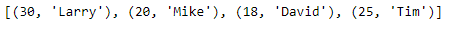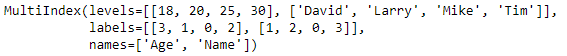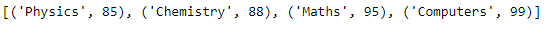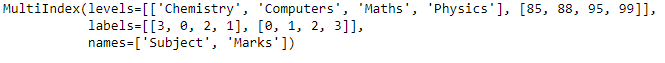# Python | Pandas MultiIndex.from_tuples()

• Last Updated : 24 Dec, 2018

Python is a great language for doing data analysis, primarily because of the fantastic ecosystem of data-centric python packages. Pandas is one of those packages and makes importing and analyzing data much easier.

Pandas` MultiIndex.from_tuples()` function is used to convert list of tuples to MultiIndex. It is one of the several ways in which we construct a MultiIndex.

Syntax: MultiIndex.from_tuples(tuples, sortorder=None, names=None)

Parameters :
tuples : Each tuple is the index of one row/column.
sortorder : Level of sortedness (must be lexicographically sorted by that level)

Returns: index : MultiIndex

Example #1: Use `MultiIndex.from_tuples()` function to construct a MultiIndex using python tuples.

 `# importing pandas as pd``import` `pandas as pd`` ` `# Creating the Tuple``tuples ``=``[(``30``, ``'Larry'``), (``20``, ``'Mike'``),``         ``(``18``, ``'David'``), (``25``, ``'Tim'``)]`` ` `# Print the Tuple``print``(tuples)`

Output :Now let’s create the MultiIndex using the Tuples.

 `# Creating the MultiIndex``midx ``=` `pd.MultiIndex.from_tuples(tuples, names ``=``(``'Age'``, ``'Name'``))`` ` `# Print the MultiIndex``print``(midx)`

Output :As we can see in the output, the function has created a MultiIndex object using the Tuples.

Example #2: Use `MultiIndex.from_tuples()` function to construct a MultiIndex using python tuples.

 `# importing pandas as pd``import` `pandas as pd`` ` `# Creating the Tuple``tuples ``=``[(``'Physics'``, ``85``), (``'Chemistry'``, ``88``),``           ``(``'Maths'``, ``95``), (``'Computers'``, ``99``)]`` ` `# Print the Tuple``print``(tuples)`

Output :Now let’s create the MultiIndex using the Tuples.

 `# Creating the MultiIndex``midx ``=` `pd.MultiIndex.from_tuples(tuples, names ``=``(``'Subject'``, ``'Marks'``))`` ` `# Print the MultiIndex``print``(midx)`

Output :My Personal Notes arrow_drop_up